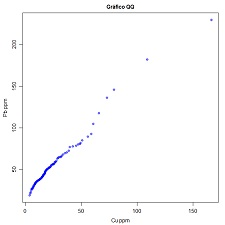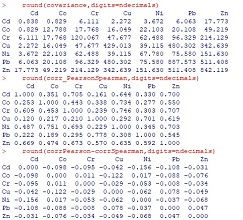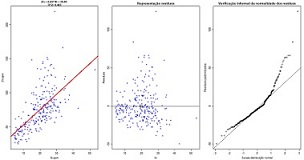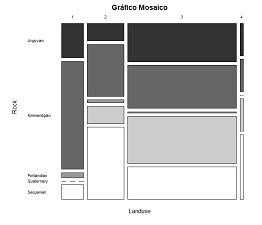## 4. Bivariate data analysis

 4.1 Scatterplot (download) Plot a scatterplot of two quantitative variables. Variables to customize: `varlistindex <- c(5,6) # index of the two GTD frame quantitative variablestitleGRAPH <- "Diagrama de dispersão" # title of the plottitleUNITS <- c("ppm","ppm") # units of the variables to add to axis legends`4.2 Scatterplot matrix (download) Plot a matrix of scatterplots of several quantitative variables. Histograms are plotted in the diagonal of the matrix. Variables to customize: `varlistindex <- c(5:11) # indexes of the several GTD frame quantitative variablesnclassesHIST <- 10 # number of classes for the histograms in the diagonalsizePOINTS <- 0.8 # size of the points in the plotssmooth <- FALSE # option (TRUE or FALSE) to interpolate density of points in each plottitleGRAPH <- "Matriz de diagramas de dispersão" # title of the graphic`4.3 Contingency table and 3D histogram of two quantitative variables (download) Compute a contingency table for equal size intervals of two quantitative variables (absolute frequencies) and plot a 3D histogram. Variables to customize:  `varlistindex <- c(5,6) # index of the two GTD frame quantitative variablesnclasses <- c(10,12) # number of classses of the two selected variablestitleUNITS <- c("ppm","ppm") # units of the variables to add to axis legendsspaceBARS <- 0.5 # space between 3D barsangles <- c(45,45) # azimuth and dip angle of the graphic, change point of viewtitleGRAPH <- "Histograma 3D" # title of the graphic`4.4 QQ plot (download) Plot a QQ plot of two quantitative variables. Variables to customize:  `varlistindex <- c(8,10) # index of the two GTD frame quantitative variablesp_spacing <- 0.01 # spacing of the percentiles to build thew QQ plottitleUNITS <- c("ppm","ppm") # units of the variables to add to axis legendsplotlineminmax <- FALSE # add a reference line between the first and the last point  titleGRAPH <- "Gráfico QQ" # title of the graphic`4.5 Matrix of QQ plots (download) Plot a matrix of QQ plots of several quantitative variables. Variables to customize: `varlistindex <- c(5:11) # indexes of the GTD frame quantitative variablesndecimals <- 3 # number of decimal places `4.6 Matrices of covariance, Pearson and Spearman correlation and diferences (download) For a set of quantitative variables, compute and display a matrix of covariance, a matrix of correlations (Pearson in the lower part and Spearman in the upper part) and a matrix of differences between Pearson and Spearman correlation coeficients. Variables to customize: `varlistindex <- c(5:11) # indexes of the GTD frame quantitative variablesndecimals <- 3 # number of decimal places`4.7 Simple linear regression (download) Fit a linear regression (Y=aX+b) between two quantitative variables (left graphic) and display equation and R2, plot residuals vs the primary variable of the regression (central graphic) and plot residuals vs a normal distribution law (informal test for residuals normality). Variables to customize: `varlistindex <- c(9,11) # index of the two GTD frame quantitative variablestitleUNITS <- c("ppm","ppm")# units of the variables to add to axis legends`4.8 Contingency table and 3D histogram of two qualitative variables  (download) Compute a contingency table for two qualitative variables (relative frequencies) and plot a 3D histogram. Variables to customize:  `varlistindex <- c(5,6) # index of the two GTD frame qualitative variablesndecimals <- 3 # number of decimal placesspaceBARS <- 0.25 # space between 3D barsangles <- c(225,45) # azimuth and dip angle of the graphic, change point of viewtitleGRAPH <- "Histograma 3D" # title of the graphic`4.9 Mosaic diagram (download) Plot a mosaic diagram of two qualitative variables. Variables to customize:  `varlistindex <- c(3,4) # index of the two GTD frame quantitative variables. Different order of the qualitative variables generate different graphics.ndecimals <- 5 # number of decimalstitleGRAPH <- "Gráfico Mosaico" # title of the graphic`4.10 Conditional cumulative histograms (download) Plot cumulative histograms of several quantitative variables conditional to the modalities of a qualitative variable. Variables to customize: `varsepindex <- 4 # index of the qualitative GTD frame variablevarlistindex <- c(5:11) # indexes of the several GTD frame quantitative variablestitleGRAPH <- "Função de distribuição cumulativa condicional" # title of the plotpar(mfrow=c(3,3),mar=c(3.5, 3.5, 2, 1), oma=c(0,0,2,0), mgp=c(2.25, 0.8, 0))# options to subdivide the graphical window, the numbers 3,3 represents rows and columns of the matrix of graphics`4.11 Conditional box-plots (download) Plot box-plots of several quantitative variables conditional to the modalities of a qualitative variable. Variables to customize: `varsepindex <- 4 # index of the qualitative GTD frame variablevarlistindex <- c(5:11) # indexes of the several GTD frame quantitative variablesplotlog <- FALSE # (TRUE or FALSE) use or not log scaletitleGRAPH <- "Box-plots condicionais" # title of the plotpar(mfrow=c(3,3),mar=c(3.5, 3.5, 2, 1), oma=c(0,0,2,0), mgp=c(2.25, 0.8, 0))# options to subdivide the graphical window, the numbers 3,3 represents rows and columns of the matrix of graphics`4.12 Conditional basic statistics (download) Compute conditional basic statistics (mean, standard deviation and skewness) of a quantitative variable conditional to the modalities of a qualitative variable. Variables to customize:  `varsepvarindex <- 4 # index of the qualitative GTD frame variablevarlistindex <- 5 # index of the quantitative GTD frame variablendecimals <- 5 # number of decimal places`